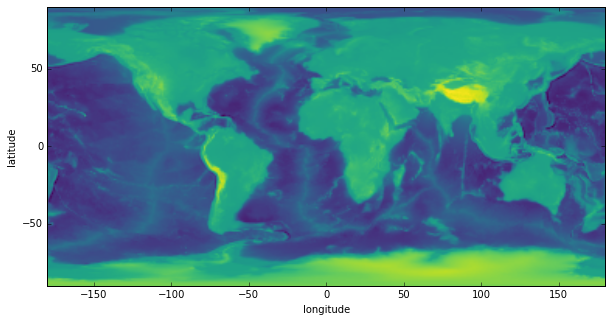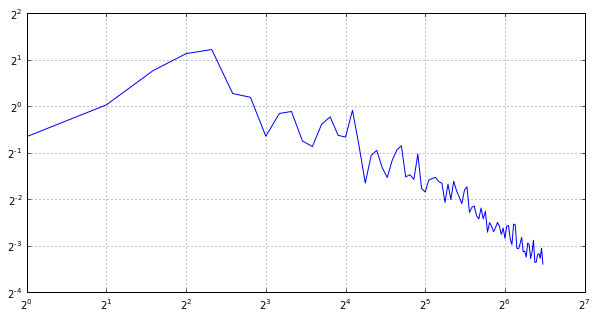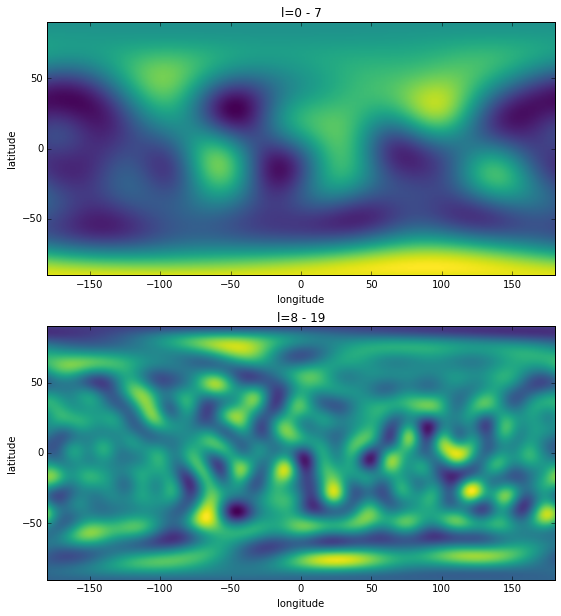# Simple Spherical Harmonic Expansions¶

This script demonstrates how to expand and analyse global data on the sphere using spherical harmonic functions.

In :
%matplotlib inline
import matplotlib.pyplot as plt
import numpy as np


In this example, we will use the topography of Earth that is provided in the example directory.

In :
# Read the gridded Earth topography data from the example directory.
infile = '../ExampleDataFiles/topo.dat.gz'
topo = np.loadtxt(infile)

# Plot the data using matplotlib.
fig, ax = plt.subplots(1, 1, figsize=(10, 5))
ax.imshow(topo, extent=(-180, 180, -90, 90), cmap='viridis')
ax.set(xlabel='longitude', ylabel='latitude');## Compute the power spectrum¶

Power spectra show how the variance of the function is distributed as a function of spherical harmonic degree. Concentration of energy (power) in spherical harmonics with a particular wavelength can give hints about the scales of the features.

In :
# Import the required functions. Alternatively, and for convenience,
# these could all be imported from the submodule pyshtools.shtools instead.
from pyshtools.expand import SHExpandDH;
from pyshtools.spectralanalysis import SHPowerSpectrumDensity;

coeffs = SHExpandDH(topo, sampling=2)
nl = coeffs.shape
ls = np.arange(nl)

# A spherical harmonic power spectrum can be calculated using different
# conventions. SHTOOLS defines the power spectrum density as the power per
# coefficent, where the power is assumed to be distributed isostropically
# among all the angular orders at degree l. This is analogous to the power
# per kx and ky in Fourier analyses of 2D functions.
power_per_lm = SHPowerSpectrumDensity(coeffs)

# The power per degree l is somewhat equivalent to the power at a given
# magnitude |k| in the 2D Fourier domain. This can be calculated using the
# function SHPowerSpectrum(coeffs), but it is equivalent to multiplying
# the power per coefficient by (2*l + 1).
power_per_l = power_per_lm * (2 * ls + 1)

# The power per log(l) 'band power' corresponds to the full coefficient
# energy in a logarithmic band (e.g., a factor 2) and is useful to analyse
# dominant scales. It is connected to localized, as opposed to standing
# wave, energy and is thus appropriate when looking at the variance spectrum
# of Earth's topography.
power_per_logl = np.log(2) * ls * power_per_l

# Plot the band power.
fig, ax = plt.subplots(1, 1, figsize=(10, 5))

ax.plot(ls, power_per_logl)
ax.set_yscale('log', basey=2)
ax.set_xscale('log', basex=2)
ax.grid()

pyshtools 3.3-beta -- Tools for working with spherical harmonics.## Simple Filtering¶

In :
from pyshtools.expand import MakeGridDH;

# A global dataset can be filtered isostropically by multiplying
# the spherical harmonic coefficients by a degree-dependent filter.
# We demonstrate this by setting the coefficients greater or equal
# to degree 8 equal to zero.
coeffs_filtered = coeffs.copy()
lmax = 8
coeffs_filtered[:, lmax:, :] = 0.

topo_filtered = MakeGridDH(coeffs_filtered, sampling=2)

# Next, we bandpass filter the data to only retain spherical harmonic
# coefficients between 8 <= l < 20.
coeffs_filtered2 = coeffs.copy()
lmin, lmax = 8, 20
coeffs_filtered2[:, :lmin, :] = 0.
coeffs_filtered2[:, lmax:, :] = 0.

topo_filtered2 = MakeGridDH(coeffs_filtered2, sampling=2)

# Plot the filtered data.
fig, (row1, row2) = plt.subplots(2, 1, figsize=(10, 10))
row1.imshow(topo_filtered, extent=(-180, 180, -90, 90), cmap='viridis')
row1.set(xlabel='longitude', ylabel='latitude', title='l=0 - 7');
row2.imshow(topo_filtered2, extent=(-180, 180, -90, 90), cmap='viridis')
row2.set(xlabel='longitude', ylabel='latitude', title='l=8 - 19');In [ ]: